# Member Articles

This forum is for user/member-created articles across the entire Qlik portfolio. Any member that is logged in can create and publish an article here. You must choose the appropriate label in order for the articles to be properly categorized.

Announcements
Skip the ticket, Chat with Qlik Support instead for instant assistance.

# Missing Manual - Top() and Bottom()

cancel
Showing results for
Did you mean:Master III

## Missing Manual - Top() and Bottom()Have you ever wondered how the examples from the Qlikview help may look like?

Please see below and enjoy responsibly...

Top() and Bottom()Description

Returns the value of expression evaluated with the chart's dimension values as they appear on the last row of the current column segment in a table or, in the case of bitmap charts, in the chart's straight table equivalent.

If the chart is one-dimensional or if the expression is preceded by the total qualifier, the current column segment is always equal to the entire column.

If the table or table equivalent has multiple vertical dimensions, the current column segment will include only rows with the same values as the current row in all dimension columns except for the column showing the last dimension in the inter field sort order. The inter field sort order for pivot tables is defined simply by the order of the dimensions from left to right. For other chart types this can be manipulated in the Chart Properties: Sort dialog. ...• Returns value from  first (Top) or last (Bottom) row(s) per each group

Syntax: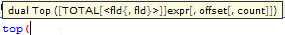TOTAL[<fld{,fld}>]]expr - expression

offset - if greater that 0 will move the evaluation of expression to rows further down or above

count - this parameter will only works with Chart Range Function (like RangeSum), it will specify the numbers of rows to be taken for calculations.(Please see last example)

Data Model:

(Copy and Pasted below code into Edit Script window and reload)

[

Year ,Month ,Sales

2015 ,January, 10

2015, February,20

2015 ,March ,30

2014 ,January ,40

2014 ,February,50

2014 ,March ,60

2013 ,January ,70

2013 ,February ,80

2013 ,March,90

]

Let's build a Straight Table with Year and Month as dimensions and expressions as below:

Example 1:

The left hand side shows use of sum(Sales) the right hand side result from our new expression.

sum( Sales )    -----------> bottom(sum( Sales ) )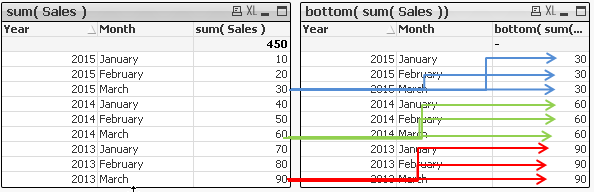For each row the returned value is the same as the bottom value for this group: 30,60.90

sum( Sales )    ----------->  top(sum( Sales ) )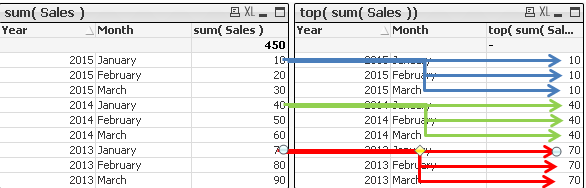For each row the returned value is the same as the bottom value for this group: 10,40,70.

Example 2
By specifying the second criteria as 2 , the returning value is the second value from the top or bottom of each group.

In this example top and bottom are giving the same result (the middle value) as we have 3 data points for each group

sum( Sales )-----------> top sum( Sales ), 2 )sum( Sales )-----------> bottom( sum( Sales ), 2 )Example 3

top(TOTAL sum( Sales ) )      bottom(TOTAL sum( Sales ) )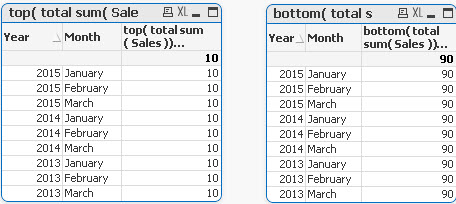Adding TOTAL before Sum will result with the first or last value to be assigned to all rows.(Total will ignore dimension)

Example 4

RangeAvg() takes 3 parameters-

expression ---> Top/Bottom(sum(Sales),

-offset of rows--->1-

number of rows to sum--->2

sum(Sales)                          rangeavg (Top(sum(Sales),1,2))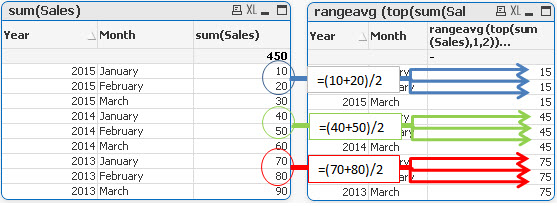For each group in right table:

• Each row is average of values from two top rows for each group (first we are moving 1 row above current row and then adding 2 rows)

sum(Sales)                          rangeavg (Bottom(sum(Sales),1,2))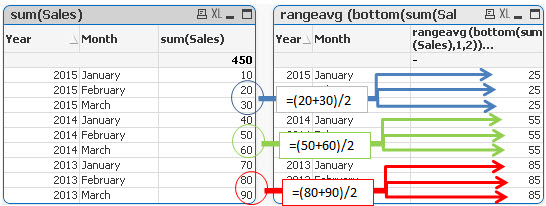For each group in right table:

• Each row is average of values from two bottom rows for each group (first we are going 1 row down current  rowand then adding 2 rows)

Still feeling hungry?

How to use - Only()

Do you Qualify?- How to use QUALIFY statement

How to use - Dimensionality()

Missing Manual - GetFieldSelections() + Bonus Example

MaxString &amp; MinString - How to + examples

The second dimension... or how to use secondarydimensionality()

Missing Manual - Below() and Above()

How to use- Dual()

Missing Manual - Before() and After()

How to use - MissingCount()

Missing Manual - Top() and Bottom()

Tags (5)
Labels (4)

• ### Technical Brief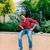Nice one